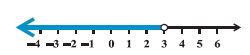Email us to get an instant 20% discount on highly effective K-12 Math & English kwizNET Programs!

#### Online Quiz (WorksheetABCD)

Questions Per Quiz = 2 4 6 8 10

### High School Mathematics - 27.11 Graphical Representation of Solutions of Inequalities

 Example 1: Solve 7x+3 < 5x+9 Solution: We have 7x+3 < 5x+9 2x < 6 or x < 3Example 2: The marks obtained by a student in his exams are 62 and 48. What marks should he get in his next test to get an average of 60? Solution: (62+8+x)/3 > = 60 x > = 70 Answer: The student must obtain a minimum of 70 marks to get an average of 60.
 Q 1: IQ of a person is given by the formula IQ = MA/CA * 100 where MA is mental age and CA is chronical age. If 80 <= IQ <= 140 for a group of 12 year children, find the range of their mental age.Less than 9.6More than 16.8Atleast 9.6 but not more than 16.8 Q 2: The longest side is of a triangle is 3 times the shortest side and third side is 2cm shorter than the longest side. If the perimeter of the triangle is atleast 61 cm, find the minimum length of the shortest side.9 cm 15 cm11 cm Q 3: x/2 < = (5x-2)/3 - (7x-3)/5Answer: Q 4: 37 - (3x+5) >= 9x-8(x-3)Answer: Q 5: The marks obtained by a student in two tests were 70 and 75, what should he score in his third test to get an average of 60?354540 Q 6: (3x-4)/2 >= (x+1)/4 -1Answer: Q 7: A solution of 8% boric acid is to be diluted by adding a 2% boric acid to it. The resulting mixture is to be more than 4%but less than 6% boric acid . If we have 640 litres of the 8% solution, how many litres of the 2% solution will have to be added?more than 320 litres but less than 1280 litresless than 3 20 litresmore than 1280 litres Q 8: A manufacturer has 6000 litres of a 12% solution of acid. How many litres of 30% acid solution must be added to it so that the acid content in the resulting mixture will be more than 15% but less than 18%?120120>x<300120>x>300 Question 9: This question is available to subscribers only! Question 10: This question is available to subscribers only!

#### Subscription to kwizNET Learning System offers the following benefits:

• Unrestricted access to grade appropriate lessons, quizzes, & printable worksheets
• Instant scoring of online quizzes
• Progress tracking and award certificates to keep your student motivated
• Unlimited practice with auto-generated 'WIZ MATH' quizzes